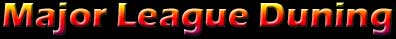General Conversions

 Mass lb = kg
 TORQUE (Ft/Lbs to Nm's) Ft/Lbs = Kg·m = N·m
 PRESSURE (Boost) in H2O = in Hg = mm Hg = PSI = atm = kG/cm2 (at) = KPA
 POWER (Horsepower to Kilowatts) HP = metric hp = KW's
 VELOCITY (MPH to KPH) ft/min = MPH = m/min = KMH  = m/s